# How to prove the normal distribution tail inequality for large x ?

• MHB
• WMDhamnekar
The last omitted term was computed by the author by using the following formula:$$\frac{1}{2}(-\frac{1}{2}\ln{x})$$

#### WMDhamnekar

MHB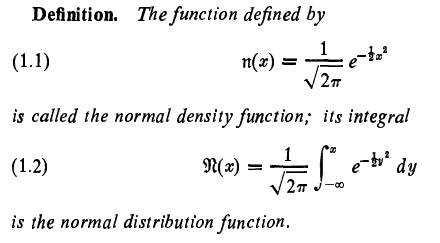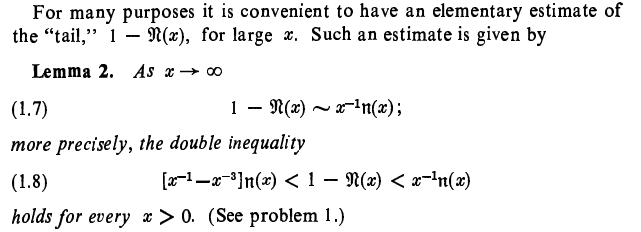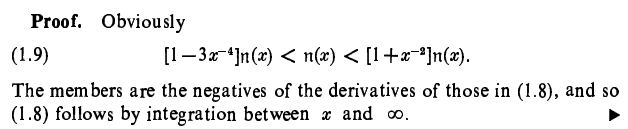What is the meaning of this proof? What is the meaning of last statement of this proof? How to prove lemma (7.1)? or How to answer problem 1 given below?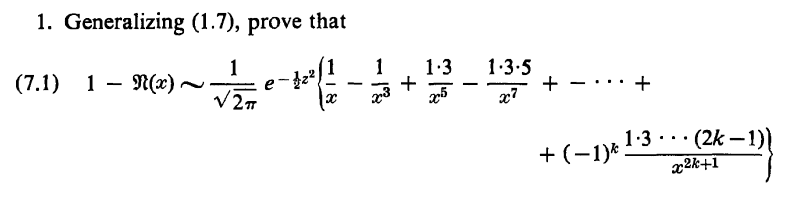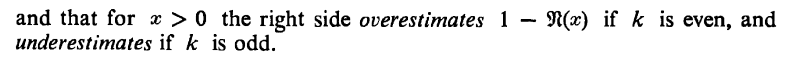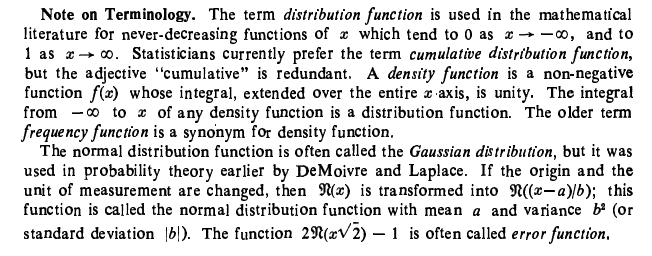Last edited:
Dhamnekar Winod said:
View attachment 11392
View attachment 11393

What is the meaning of this proof? What is the meaning of last statement of this proof? How to prove lemma (7.1)? or How to answer problem 1 given below?

If $x>0$ then the inequality in (1.9) must be true, because the left side is then slightly less than n(x), and the right side is slightly more than n(x).

Let's consider the derivatives of the expressions in (1.8).
$$\frac d{dx}(1-\Re(x)) = -\Re'(x) = -n(x)$$

Let's first find $n'(x)$.
We have:
$$n'(x)=\frac d{dx} \frac 1{\sqrt{2\pi}} e^{-\frac 12x^2} = \frac 1{\sqrt{2\pi}} e^{-\frac 12x^2} \cdot -x =-xn(x)$$
Then we have for instance:
$$\frac d{dx}(x^{-1}n(x)) = -x^{-2}n(x) + x^{-1}n'(x) = -x^{-2}n(x)+x^{-1}\cdot -x n(x) = -(1+x^{-2})n(x)$$
So we see that the derivatives of the expressions in (1.8) are indeed the negatives of the expressions in (1.9).

We have that $1-\Re(x)$ is in between 2 expressions, so its integration must also be between the integrations of the those 2 expressions.
Qed.

To prove the more general formula, we need to repeat these steps for the additional terms.

Klaas van Aarsen said:
If $x>0$ then the inequality in (1.9) must be true, because the left side is then slightly less than n(x), and the right side is slightly more than n(x).

Let's consider the derivatives of the expressions in (1.8).
$$\frac d{dx}(1-\Re(x)) = -\Re'(x) = -n(x)$$

Let's first find $n'(x)$.
We have:
$$n'(x)=\frac d{dx} \frac 1{\sqrt{2\pi}} e^{-\frac 12x^2} = \frac 1{\sqrt{2\pi}} e^{-\frac 12x^2} \cdot -x =-xn(x)$$
Then we have for instance:
$$\frac d{dx}(x^{-1}n(x)) = -x^{-2}n(x) + x^{-1}n'(x) = -x^{-2}n(x)+x^{-1}\cdot -x n(x) = -(1+x^{-2})n(x)$$
So we see that the derivatives of the expressions in (1.8) are indeed the negatives of the expressions in (1.9).

We have that $1-\Re(x)$ is in between 2 expressions, so its integration must also be between the integrations of the those 2 expressions.
Qed.

To prove the more general formula, we need to repeat these steps for the additional terms.
But, how to use this information to solve the given problem 1 or lemma 7.1?

Dhamnekar Winod said:
But, how to use this information to solve the given problem 1 or lemma 7.1?
Write (7.1) in the same form as (1.8) with the series on the left and also on the right.
Take the derivatives to find an expression that is in the same form as (1.9).
Then the proof follows in the same fashion.

Klaas van Aarsen said:
Write (7.1) in the same form as (1.8) with the series on the left and also on the right.
Take the derivatives to find an expression that is in the same form as (1.9).
Then the proof follows in the same fashion.
But I got some math help from wikipedia.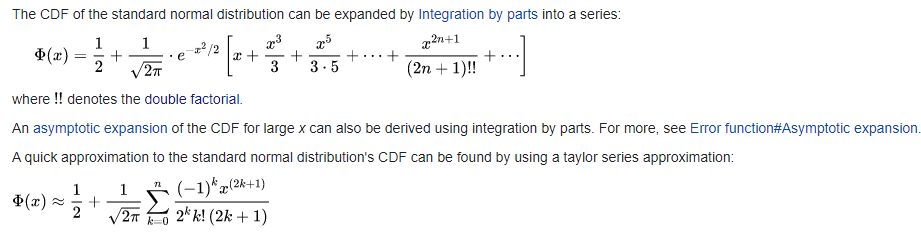How can we use the above two formulas of CDF of Normal distribution to prove lemma 7.1 in the original question?

I got the following proofs of expansion of CDF of standard normal distribution.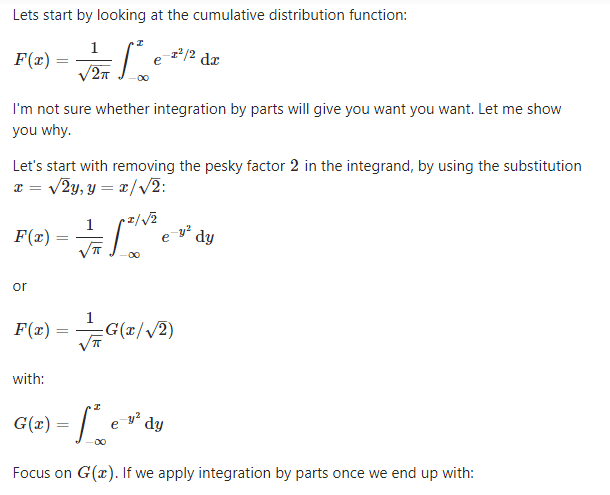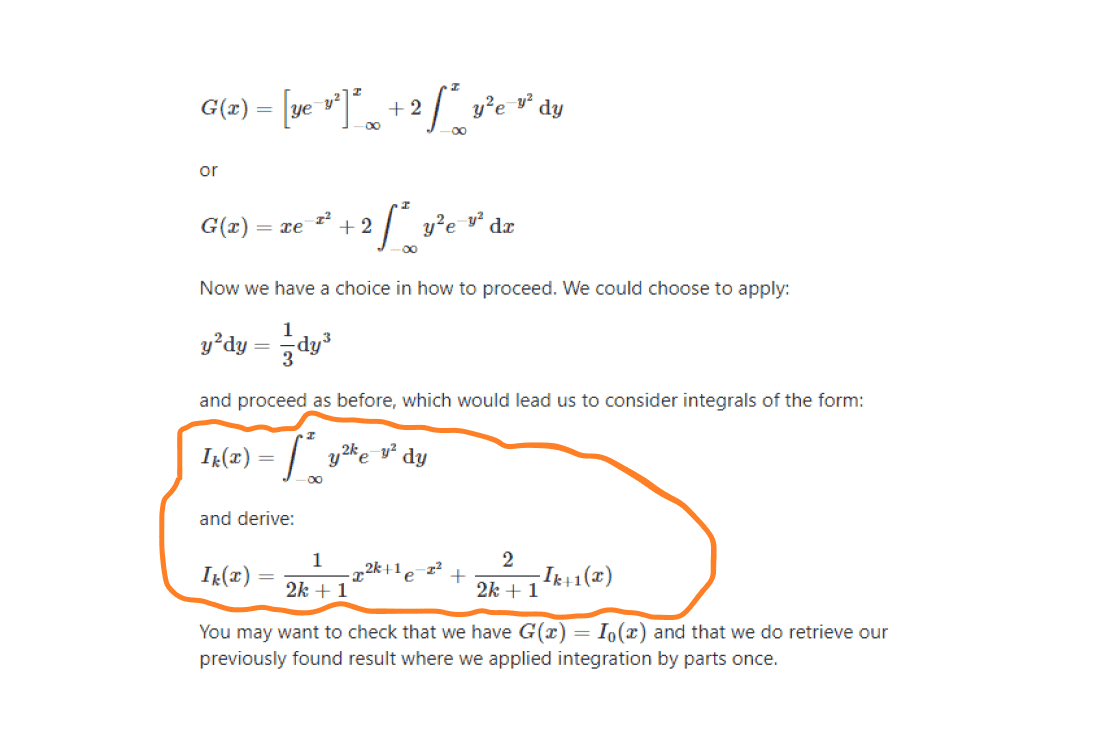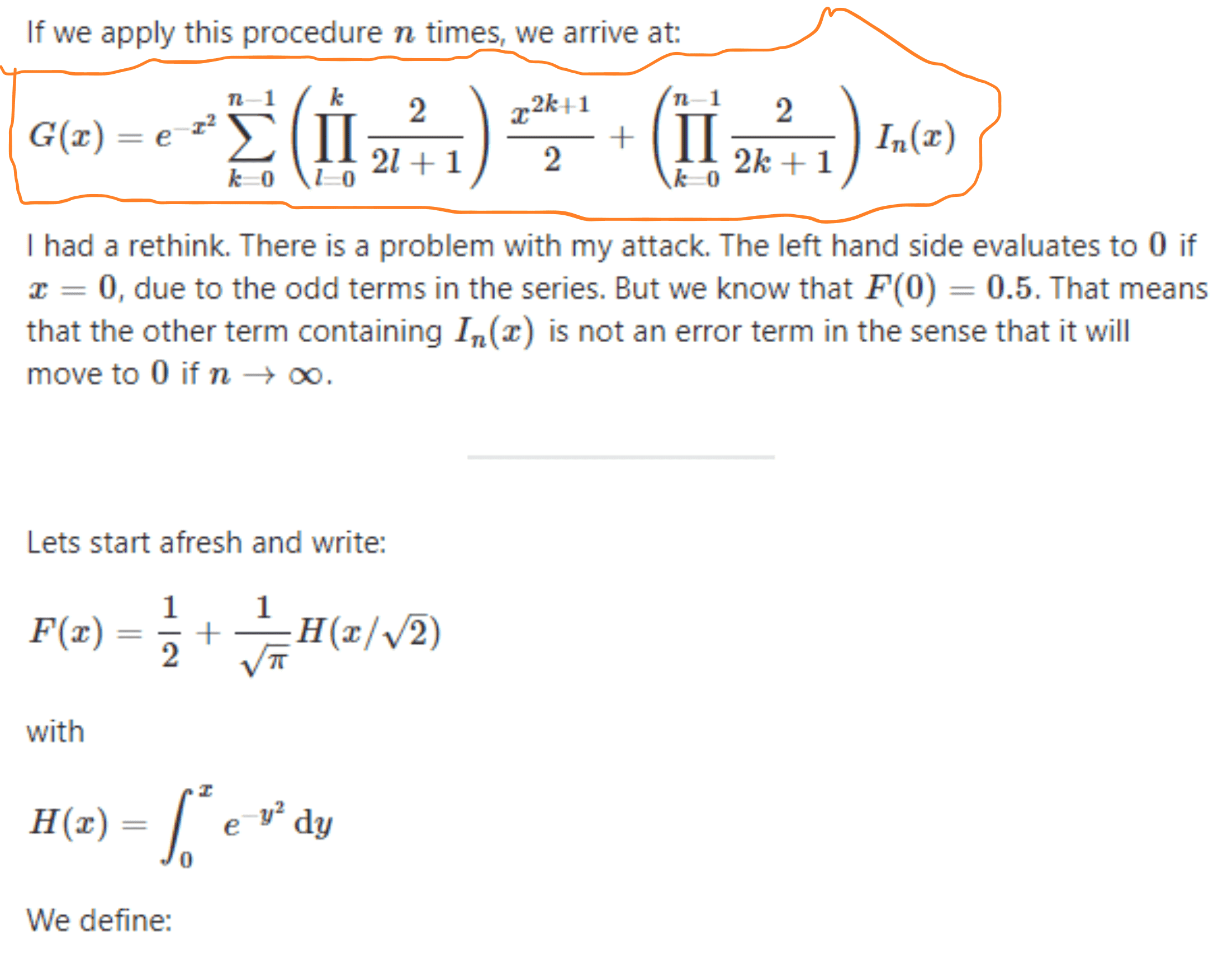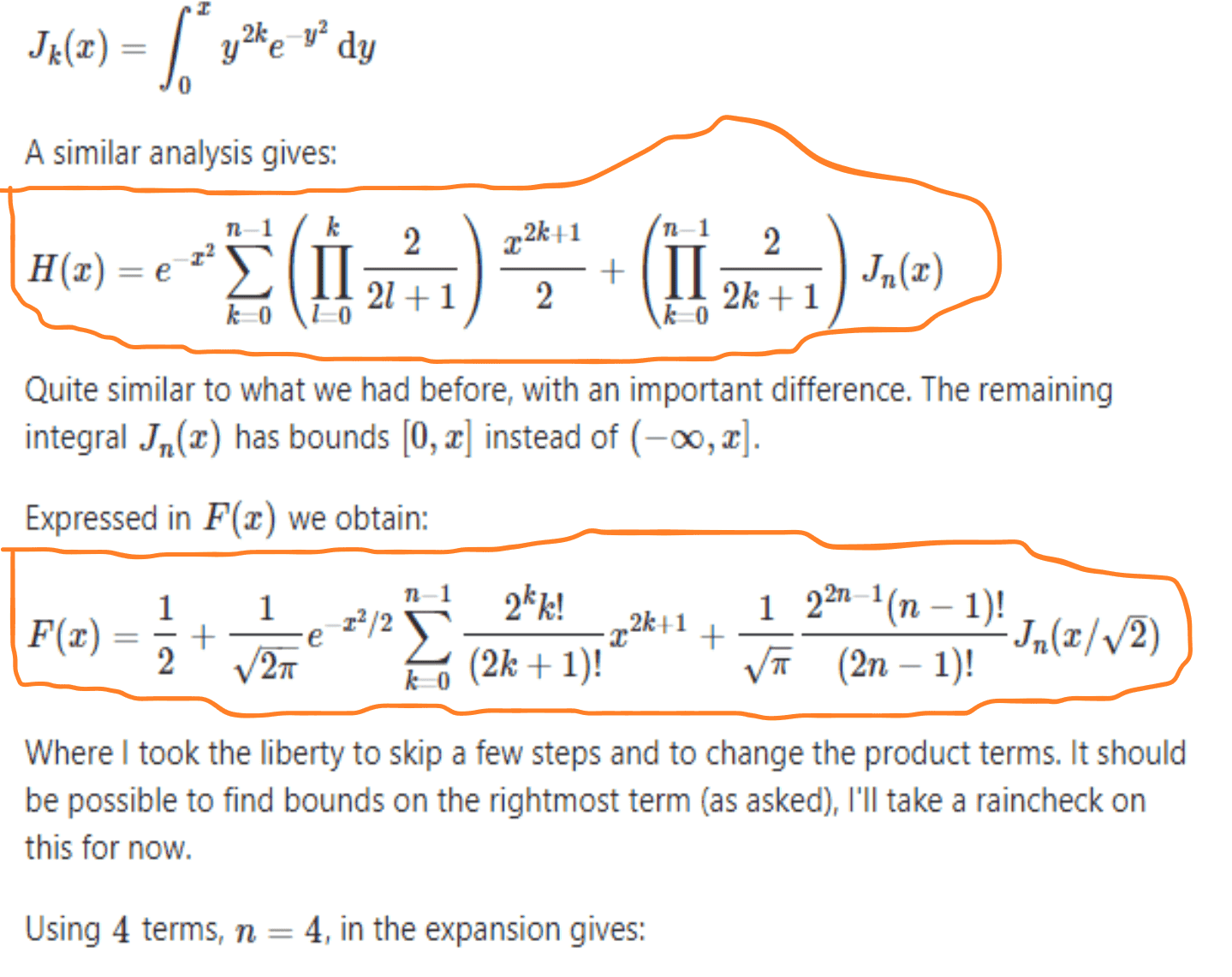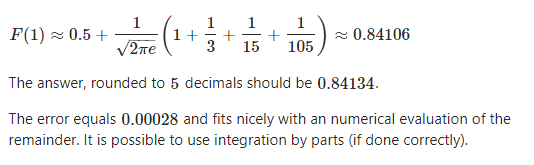In the above expansions of CDF of standard normal distribution, I want to know how the highlighted or marked computations was performed. If any member of this MHB knows the method of these computations, may explain it in reply.

Following is the simple proof: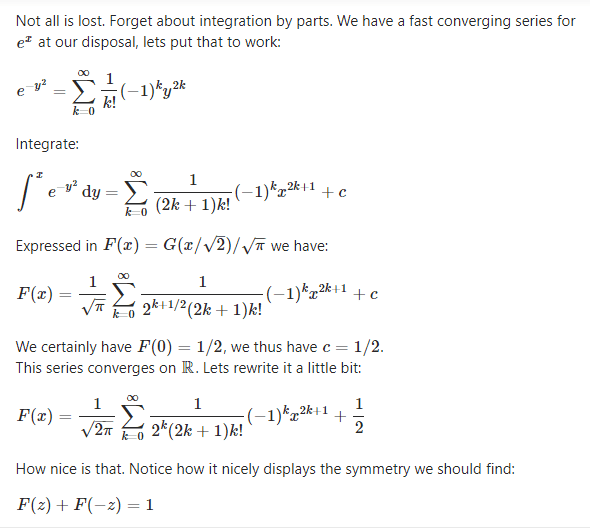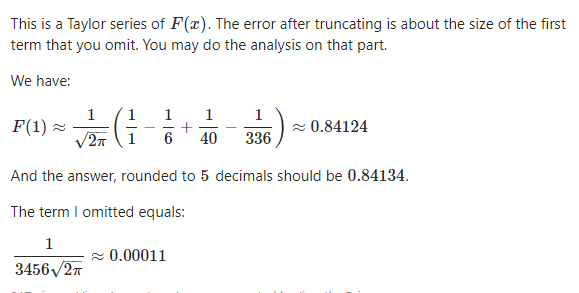How the last omitted term was computed by the author?

Last edited: